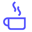a concise shot of dev knowledge
Become a Contributor
Concise shots of dev knowledge

RELATED TAGS

algorithm

# What is the DES algorithm?Edpresso Team

Data Encryption Standard (DES) is a block cipher algorithm that takes plain text in blocks of 64 bits and converts them to ciphertext using keys of 48 bits. It is a symmetric key algorithm, which means that the same key is used for encrypting and decrypting ​data.Encryption and decryption using the DES algorithm.

## Steps for generating keys

There are 16 rounds of encryption in the algorithm, and a different key is used for each round. How​ keys are generated is listed below.

Bits are labeled from 1 to 64 starting from the most significant bit and going to the least significant bit.

1. Compress and transpose the given 64-bit key into a 48-bit key using the following table:
// The array elements denote the bit numbers
int pc1 = {
57,49,41,33,25,17,9,
1,58,50,42,34,26,18,
10,2,59,51,43,35,27,
19,11,3,60,52,44,36,
63,55,47,39,31,23,15,
7,62,54,46,38,30,22,
14,6,61,53,45,37,29,
21,13,5,28,20,12,4
};
PC-1 table
1. Divide the result into two equal parts: C and D.

2. C and D are left-shifted circularly. For encryption rounds 1, 2, 9, and 16 they are left shifted circularly by 1 bit; for all of the other rounds, they are left-circularly shifted by 2.

3. The result is compressed to 48 bits in accordance with the following rule:

int pc2 = {
14,17,11,24,1,5,
3,28,15,6,21,10,
23,19,12,4,26,8,
16,7,27,20,13,2,
41,52,31,37,47,55,
30,40,51,45,33,48,
44,49,39,56,34,53,
46,42,50,36,29,32
};
PC-2 table
1. The result of step 3 is the input for the next round of key generation.

## Steps for encryption

1. Transpose the bits in the 64-block according to the following:
// 58 means that the 58th bit should be considered
// the first bit, 50th bit the second bit and so on.
int initial_permutation_table = {
58,50,42,34,26,18,10,2,
60,52,44,36,28,20,12,4,
62,54,46,38,30,22,14,6,
64,56,48,40,32,24,16,8,
57,49,41,33,25,17,9,1,
59,51,43,35,27,19,11,3,
61,53,45,37,29,21,13,5,
63,55,47,39,31,23,15,7
}; 
The initial permutation table
1. Divide the result into equal parts: left plain text (1-32 bits) and right plain text (33-64 bits)

2. The resulting parts undergo 16 rounds of encryption in each round.

The right plain text is expanded using the following expansion table:

// The array elements denote the bit numbers
int expansion_table = {
32,1,2,3,4,5,4,5,
6,7,8,9,8,9,10,11,
12,13,12,13,14,15,16,17,
16,17,18,19,20,21,20,21,
22,23,24,25,24,25,26,27,
28,29,28,29,30,31,32,1
}; 
The expansion table
1. The expanded right plain text now consists of 48 bits and is XORed with the 48-bit key.

2. The result of the previous step is divided into 8 boxes. Each box contains 6 bits. After going through the eight substitution boxes, each box is reduced from 6 bits to 4 bits. The first and last bit of each box provides the row index, and the remaining bits provide the column index. These indices are used to look-up values in a substitution box. A substitution box has 4 rows, 16 columns, and contains numbers from 0 to 15.

3. The result is transposed in accordance with the following rule:​

// The array elements denote the bit numbers
int permutation_table = {
16,7,20,21,29,12,28,17,
1,15,23,26,5,18,31,10,
2,8,24,14,32,27,3,9,
19,13,30,6,22,11,4,25
};
The permutation table
1. XOR the left half with the result from the above step. Store this in the right plain text.

2. Store the initial right plain text in the left plain text.

3. These halves are inputs for the next round. Remember that there are different keys for each round.

4. After the 16 rounds of encryption, swap the left plain text and the right plain text.

5. Finally, apply the inverse permutation (inverse of the initial permutation), ​and the ciphertext will be generated.

### Steps for decryption

The order of the 16 48-bit keys is reversed such that key 16 becomes key 1, and so on. Then, the steps for encryption are applied to the ciphertext.

RELATED TAGS

algorithm
RELATED COURSES

View all Courses

Keep Exploring

Learn in-demand tech skills in half the time